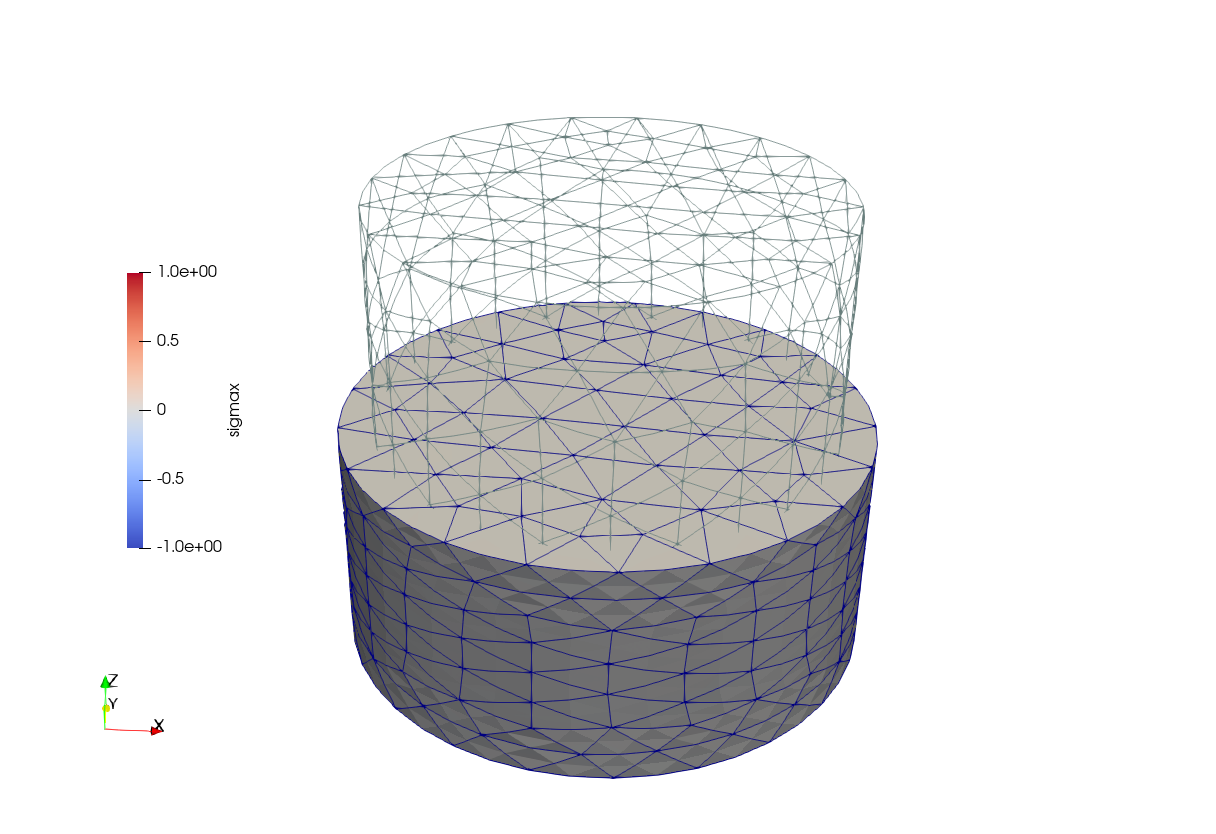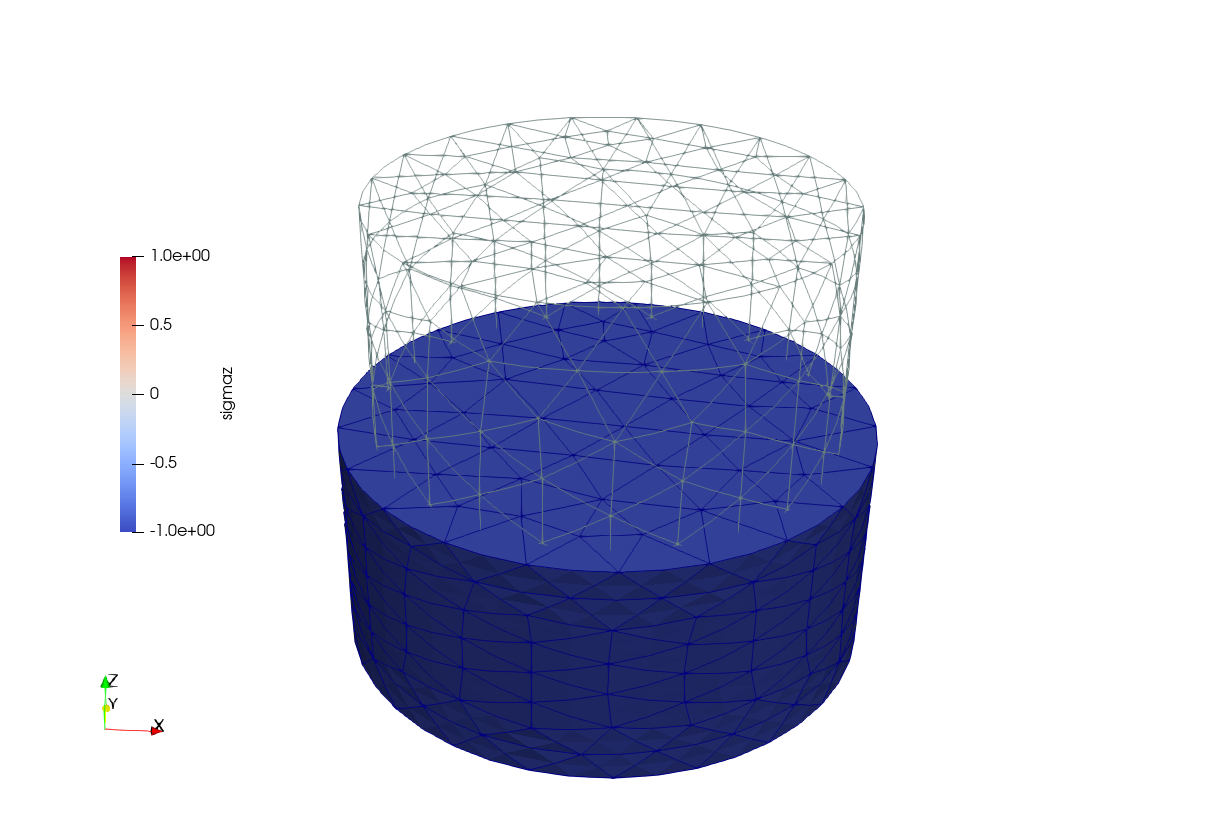# Cylinder under pure compression

 Title Cylinder under pure compression Tags elasticity compression Runnng time 1 sec See also 003-cube-pure-tension CAEplex case https://caeplex.com/p/ed77e Available in HTML PDF ePub

# 1 Problem description

A non-dimensional cylinder of radius 1/2 and height 1 whose base rests on the x-y plane centered at the origin is subject to a nondimensional compressive pressure of 1 unit in the negative z direction acting on the face at z=1 (fig. 1). The cube has a non-dimensional unitary Young modulus E=1 and Poisson’s ratio \nu=0.3. To remove rigid-body displacemetns while obtaining pure compressive stress, instead of fixing lower-dimensional entities as in Cube under pure tension, the bottom face is set to have both tangential and radial symmetry displacement conditions.

Once again, as in Cube under pure tension, the displacements and stresses distribution within the cylinder are to be obtained. The stress \sigma_x is expected to be uniformly equal to one with the other five stress components (\sigma_y, \sigma_z, \tau_{xy}, \tau_{yz} and \tau_{zx}) to be identically equal to zero. The cylinder is expected to shrink in the z direction and to expand in both the x and y directions due to the non-zero Poisson’s ratio \nu.

The mesh is expected to be fully unstructured and not optimized as to show that the expected results are recovered with the numerical calculation independently of having a mesh composed of wedges and/or hexahedra within an azimuthal array. In this case, the actual area of the discretized surface that holds the external load does depend on the mesh coarseness, with the theoretical value of \pi/4 recovered only in the limit of infinite elements. However, the load condition in this case is an uniform pressure and not a force, so the normal stress in the axial direction is expected to be numerically equal to such pressure.

# 2 Geometry and mesh

The geometry is created and meshed directly from Gmsh. Only two physical surfaces are needed for the boundary conditions, namely “bottom” and “top.” The physical volume is needed to get volumetric elements in the resulting cylinder.msh file. The mesh is unstructured tetrahedral due to the reasons already explained.

SetFactory("OpenCASCADE");

Cylinder(1) = {0, 0, 0, 0, 0, 1, 0.5};

Physical Surface("bottom") = {3};
Physical Surface("top") = {2};
Physical Volume("bulk") = {1};

Mesh.ElementOrder = 2;

# 3 Input file

This time the input file cylinder.fin is not annotated yet again it is self-explanatory. It solves the problem and writes a VTK file.

MESH FILE_PATH cylinder.msh

E = 1
nu = 0.3

PHYSICAL_GROUP top    BC P=1

FINO_STEP

MESH_POST FILE_PATH cylinder.vtk VECTOR u v w sigmax sigmay sigmaz tauxy tauyz tauzx

Note the two special boundary conditions tangential and radial. The first one restricts displacements along the local normal of the surface. The latter forces displacements within the surface plane to be radially distributed around the surface’s barycenter.

# 4 Execution

$gmsh -v 0 -3 cylinder.geo$ fino cylinder.fin
$ # 5 Results Figs. 2 (a), 2 (b) show \sigma_x and \sigma_y in the final deformed condition. Fig. 3 shows an interactive 3D view obtained by CAEplex where the effect of the radial symmetry condition can be seen when the displacements are warped with the slider.ab Figure 2: Stresses over the displaced grid and comparison with the original cube.. a — \sigma_x, b — \sigma_z Figure 3: Interactive results from CAEplex (rotate and warp it with the slider). ## 5.1 Check The \sigma_z(x,y,z) distribution is expected to be identically equal to minus one and all the other five stresses are expected to vanish. To verify this is the case, the following input file is built which includes the basic solution, finds the maximum and minimum values of all the six stresses and prints them out in the standard output. As handy as the radial keyword is to simplify the solution of symmetric problems, there are two catches a cognizant user needs to be aware of. On the one hand, this kind of boundary condition is not a traditional essential BC where the value of the unknown (in this case displacements) is set up to the solver’s tolerance, but a multi-freedom constrain which is implemented with a penalty method. In the general case, the tangential condition is also implemented as a multi-freedom constrain. Yet Fino is smart enough and detects that the outward normal is (0,0,-1) and then condition is translated into a traditional Dirichlet-type condition w=0. Hence the radial condition is approximately set, and the extent to which this setting applies depends on a number of things—including of course the penalty weight. This approximation propagates down to the results, as illustrated in the following check test, which reads both the solver’s tolerance (default 10^{-6}) and the penalty weight (default 10^8) from the command line. fino_reltol =$1
fino_penalty_weight = $2 INCLUDE cylinder.fin # compute min/max stresses MESH_FIND_MINMAX FUNCTION sigmax MIN sigmax_min MAX sigmax_max MESH_FIND_MINMAX FUNCTION sigmay MIN sigmay_min MAX sigmay_max MESH_FIND_MINMAX FUNCTION sigmaz MIN sigmaz_min MAX sigmaz_max MESH_FIND_MINMAX FUNCTION tauxy MIN tauxy_min MAX tauxy_max MESH_FIND_MINMAX FUNCTION tauyz MIN tauyz_min MAX tauyz_max MESH_FIND_MINMAX FUNCTION tauzx MIN tauzx_min MAX tauzx_max PRINT "stress\tmin\t\tmax" PRINT SEP "\t" "% +5e" "sigmax" sigmax_min sigmax_max PRINT SEP "\t" "% .5e" "sigmay" sigmay_min sigmay_max PRINT SEP "\t" "% .5e" "sigmaz" sigmaz_min sigmaz_max PRINT SEP "\t" "% .5e" "tauxy" tauxy_min tauxy_max PRINT SEP "\t" "% .5e" "tauyz" tauyz_min tauyz_max PRINT SEP "\t" "% .5e" "tauzx" tauzx_min tauzx_max  $ fino check.fin 1e-3 1e4
stress  min             max
sigmax  -1.861889e-03   +2.982465e-03
sigmay  -2.07812e-03     3.01866e-03
sigmaz  -1.00348e+00    -9.96333e-01
tauxy   -1.34599e-03     1.16549e-03
tauyz   -1.48900e-03     1.04906e-03
tauzx   -1.34727e-03     1.66753e-03
$fino check.fin 1e-3 1e12 stress min max sigmax -3.368149e-03 +1.916099e-03 sigmay -2.82702e-03 3.11235e-03 sigmaz -1.00313e+00 -9.96947e-01 tauxy -8.14560e-04 7.82537e-04 tauyz -1.44005e-03 1.47797e-03 tauzx -1.70246e-03 1.29679e-03$ fino check.fin 1e-9 1e4
stress  min             max
sigmax  -6.090733e-04   +4.732937e-04
sigmay  -7.18812e-04     5.77535e-04
sigmaz  -1.00135e+00    -9.97135e-01
tauxy   -6.64507e-04     6.27475e-04
tauyz   -1.08775e-03     1.17853e-03
tauzx   -1.13649e-03     1.02294e-03
$fino check.fin 1e-9 1e12 stress min max sigmax -3.826204e-03 +1.897524e-03 sigmay -2.78529e-03 2.49913e-03 sigmaz -1.00174e+00 -9.97135e-01 tauxy -7.08823e-04 1.06614e-03 tauyz -1.08773e-03 1.17850e-03 tauzx -1.65213e-03 1.02282e-03$

On the other hand, the imposition of the radial symmetry does depend on the mesh size (take a minute and think about it!). In effect, the input file check-fine.fin solves the same proble as check.fin with a finer mesh. For the default values of the tolerance and the weight, we get

$fino check.fin 1e-6 1e8 stress min max sigmax -6.090465e-04 +4.738302e-04 sigmay -7.17874e-04 5.77485e-04 sigmaz -1.00135e+00 -9.97133e-01 tauxy -6.65185e-04 6.28188e-04 tauyz -1.08804e-03 1.17926e-03 tauzx -1.13719e-03 1.02356e-03$ fino check-fine.fin 1e-6 1e8
stress  min             max
sigmax  -1.360267e-04   +1.208525e-04
sigmay  -1.17141e-04     1.03318e-04
sigmaz  -1.00021e+00    -9.99558e-01
tauxy   -1.01711e-04     1.00290e-04
tauyz   -2.23632e-04     2.59015e-04
tauzx   -2.37351e-04     2.68281e-04
\$ 

The conclusion is that this kind of “handy” constrain limits the precision of the stresses both through the weight of the penalty method and through the mesh size, although it is still overall good (\sim \pm 1\%) for a rather coarse mesh. Recall that in Cube under pure tension the results only depended on the precision of the linear solver. Once again, if we passed the --mumps option, the results would not depend on the first argument but they would still depend on the second one.